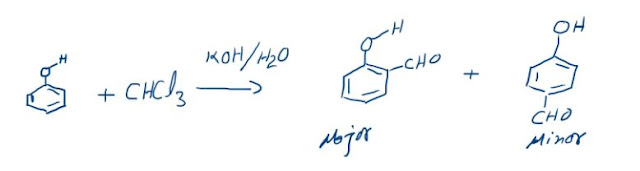### STATE OF A GAS:

The term “state” has many different meanings in chemistry. We already have met one meaning while writing “states of matter”. The second meaning is physical condition of a system, in this case, the Ideal gas. To define the physical condition of a gas there are certain variables called “State Variables”. There are majorly three state variables for a given sample of gas: Pressure, Volume and Temperature (P, V and T). When these three values are fixed for gas we say that the gas is in a fixed state and when value of any of the state variable changes the state of the gas also changes.
Change of State: P1 V1 T1  ---> P2 V2 T2 (From state 1 to state 2) The amount of a gas is calculated in terms of number of moles, n (mass/molar mass).
PRESSURE:
The molecular collisions of the gas, with the walls of the container or anything with which it comes in contact, results in force being applied. This force averaged per unit area is called “Pressure”.
P = Force / Area
The SI units of pressure are N/m2 or Pascal (Pa). There are many other units used for pressure. They are as under:
Atmosphere (atm), Bar, mm/cm Hg column, Torr

IDEAL GAS LAWS:
These laws relate the states of a gas through the various state variables. That is, how the state variables are related to each other.
1. Boyle’s Law: relates P and V at constant T for a given sample.
2. Charle’s Law: relates V and T at constant pressure for a given sample.
3. Gay-Lussac’s Law: relates P and T at constant volume for a given sample.
4. Avogadro’s Principle: relates V and n at constant P and T.
Then we will combine all these laws to obtain the combined equation of state.

### The Reimer-Tiemann reaction: Reaction and its Mechanism

Reimer-Tiemann Reaction Phenols on reaction with chloroform in the presence of sodium hydroxide (or potassium hydroxide) solution give hydro...